# Aggregate Functions in Tableau

• Difficulty Level : Expert
• Last Updated : 24 Oct, 2020

• Tableau: Tableau is a very powerful data visualization tool that can be used by data analysts, scientists, statisticians, etc. to visualize the data and get a clear opinion based on the data analysis. Tableau is very famous as it can take in data and produce the required data visualization output in a very short time.
• Aggregate Function: Aggregate function can be a function where the values ​​of multiple lines are grouped together to form a single summative value. Typical integration functions include: Measurement (e.g., arithmetic means), Count, etc.

In a Tableau, you will combine steps or sizes, although it is very common to combine steps. Whenever you add a rating to your view, the aggregation is used when the rating is automated, the type of aggregation used varies by counting in viewing context. Tableau offers a variety of integrated functions, which help to make aggregations such as calculating total, average, minimum, quantity, etc. between this article, we will show you ways to use the functions of Tableau Aggregate with examples.

The most commonly used functions in tableau are listed below:

1. SUM: sum of values
2. AVG: average of values
3. MIN: minimum of values
4. MAX: maximum of values
5. VAR: variance of sample population
6. VARP: variance of entire population
7. STDEV: standard deviation of sample population
8. STDEVP: standard deviation of entire population
9. COUNT: count of values

### Tableau Sum Function

The Tableau Sum function is employed to seek out the Sum of records during a column.

Syntax:

```SUM(Expression)
```

Example:

To demonstrate these Tableau aggregate functions, we’ve to use the Calculated Field. Please navigate to Analysis Tab and choose the Create Calculated Field (choice to create a calculated field and use sum aggregate function).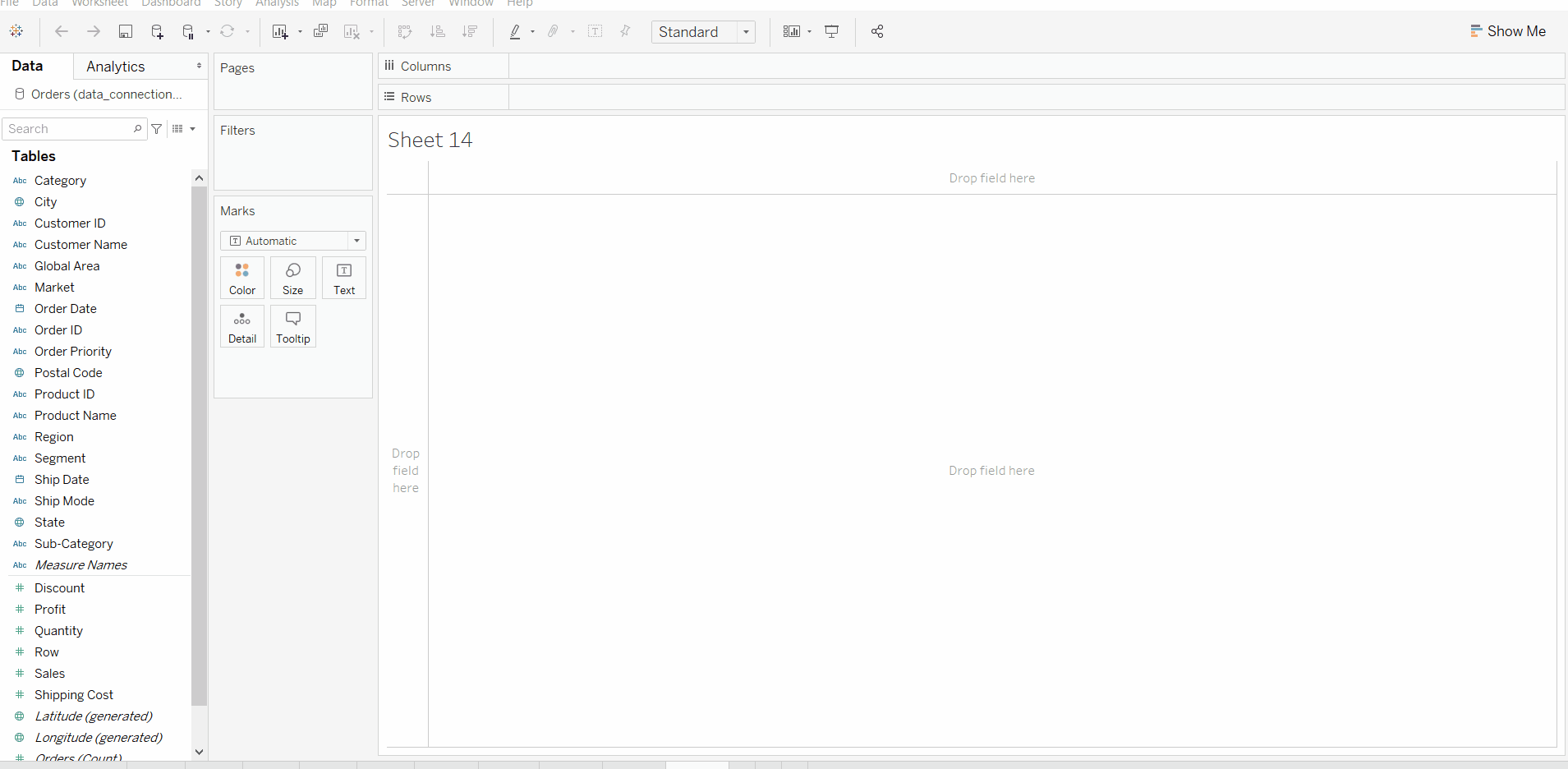### Tableau Avg Function

The Tableau Avg or average function is used to calculate the Average.

Syntax:

```AVG(Expression)
```

Example:

As you’ll see from the below screenshot, we are creating a replacement field (Sales Average) using Tableau function.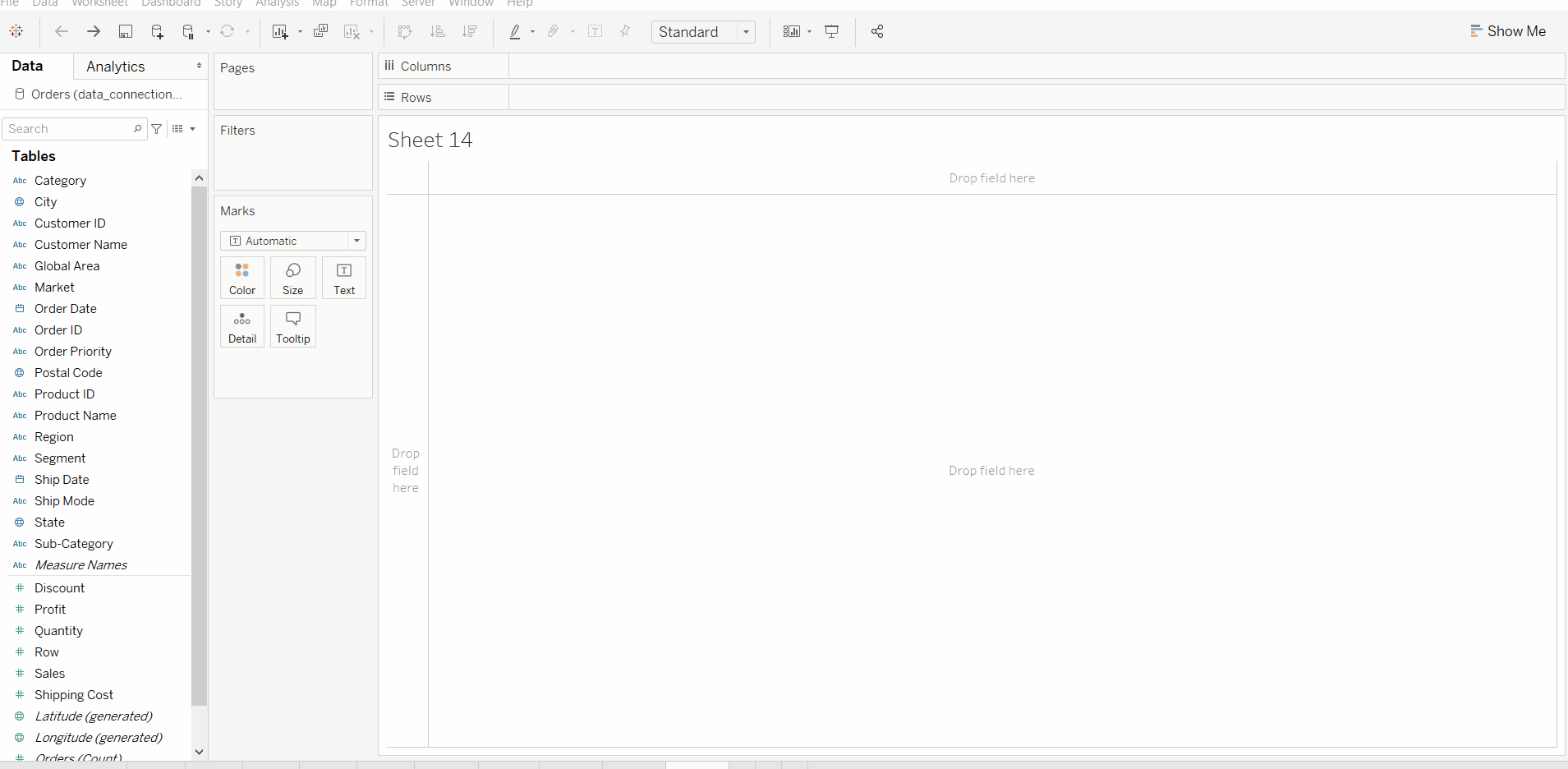### Tableau MIN Function

The Tableau MIN function is an aggregate function in Tableau, which is employed to seek out the minimum value.

Syntax:

```MIN(Expression1, Expression2)
```

Example:

The Tableau MIN function accepts two arguments. Use this to find the smallest between two numbers.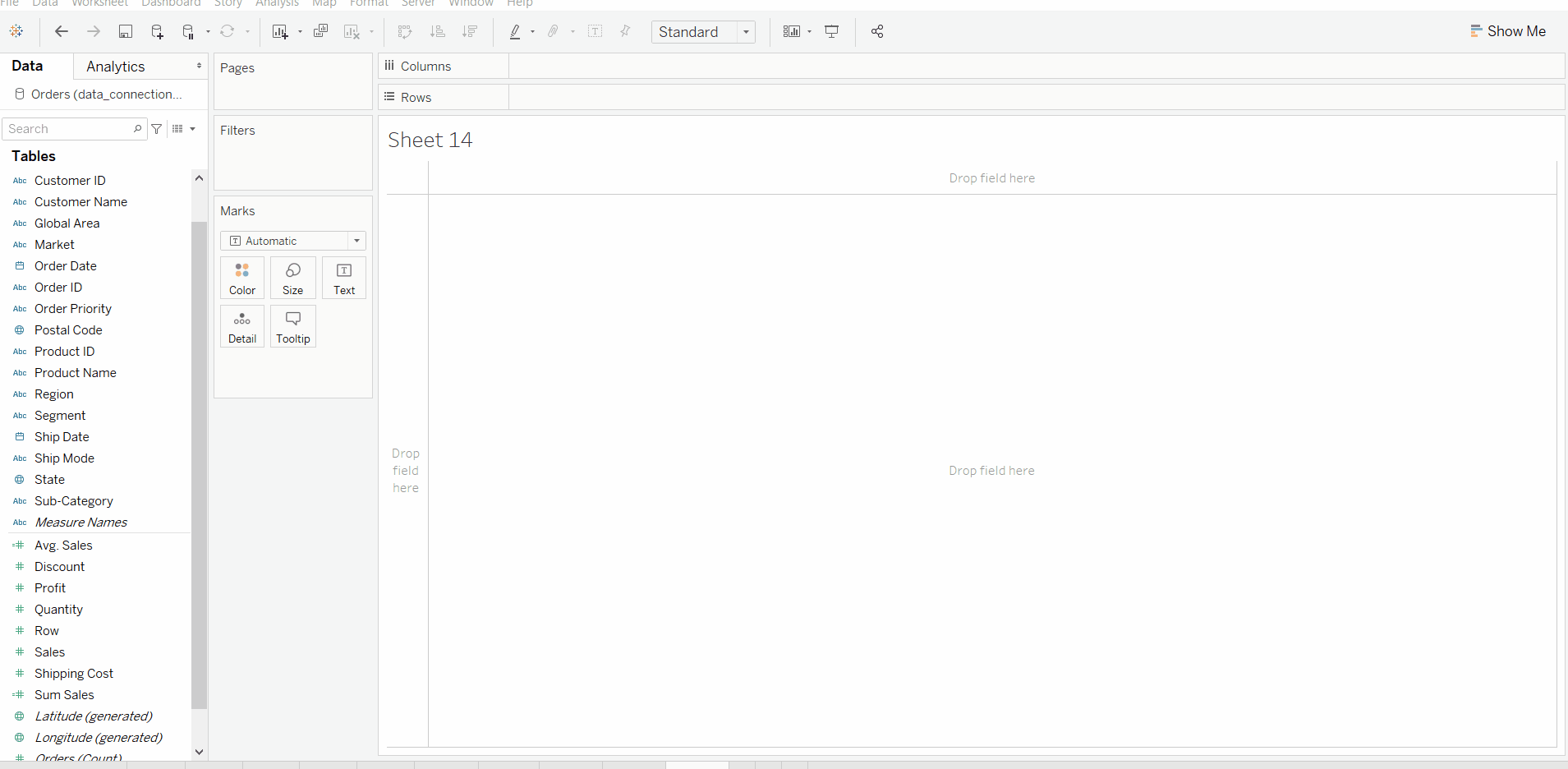### Tableau MAX Function

The Tableau MAX function is employed to seek out the utmost value.

Syntax:

```MAX(Expression 1, Expression 2)
```

Example:

In Tableau, the MAX function accepts two arguments. Use this to find the largest among two numbers.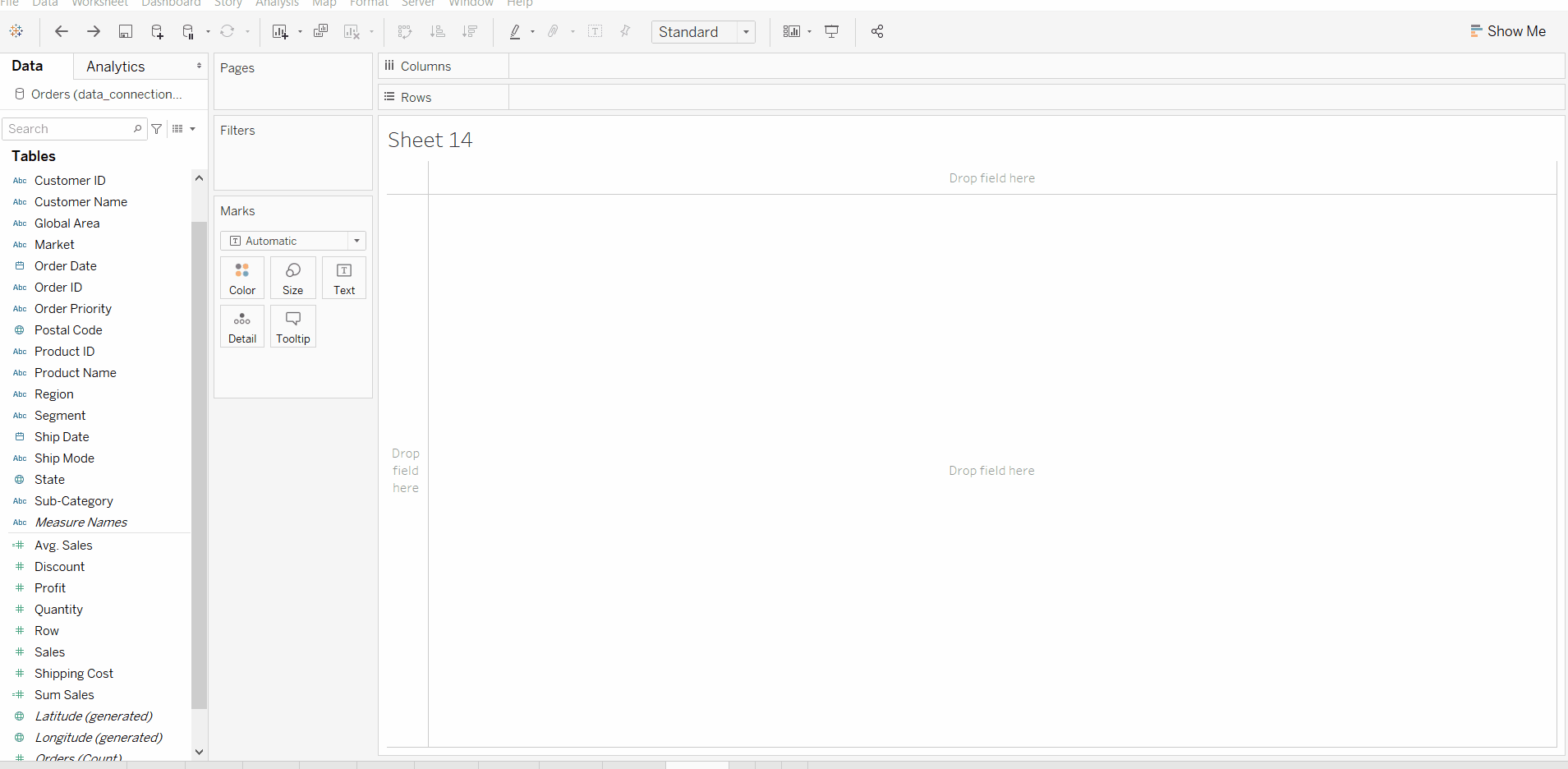### Tableau VAR Function

The Tableau VAR function is an aggregate function in tableau, which is used to find the Variance of the sample population.

Syntax:

```VAR(Expression)
```

Example: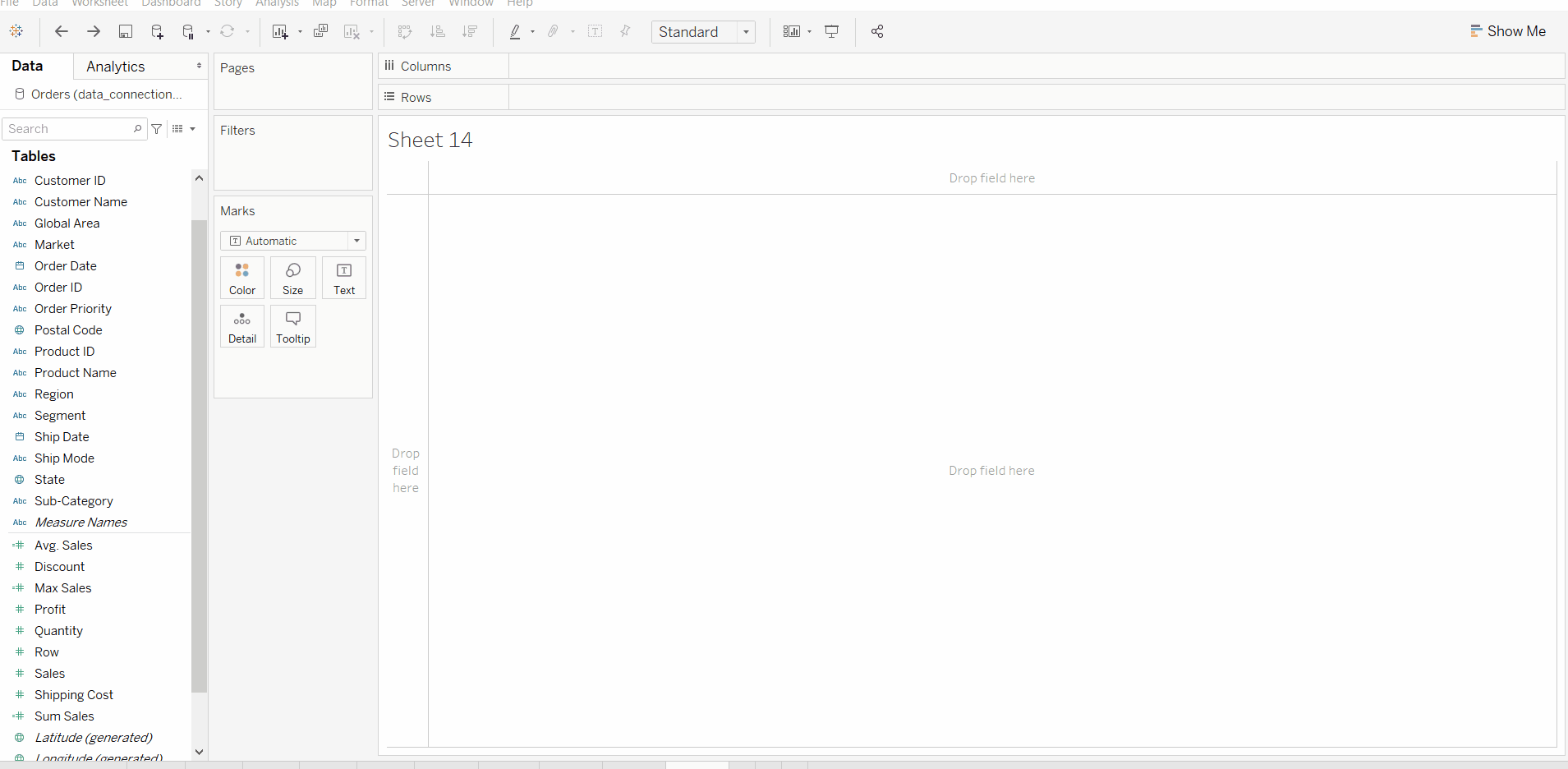### Tableau VARP Function

The Tableau VARP function is employed to seek out the Variance of the whole population.

Syntax:

```VARP(Expression)
```

Example: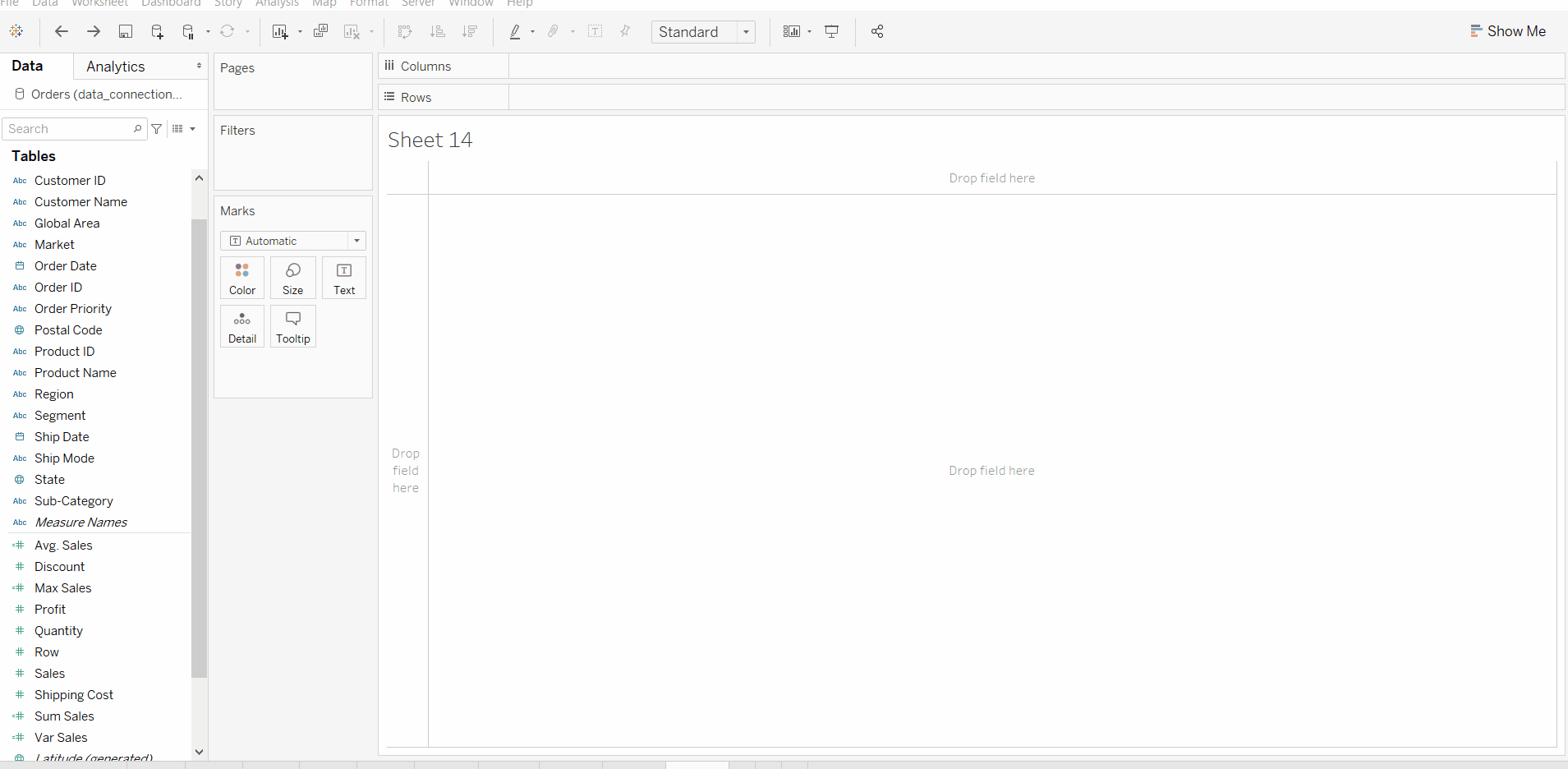### Tableau STDEV Function

The Tableau STDEV function is one of the aggregate functions in tableau, which is used to find the standard deviation of the sample population.

Syntax:

```STDEV(Expression)
```

Example: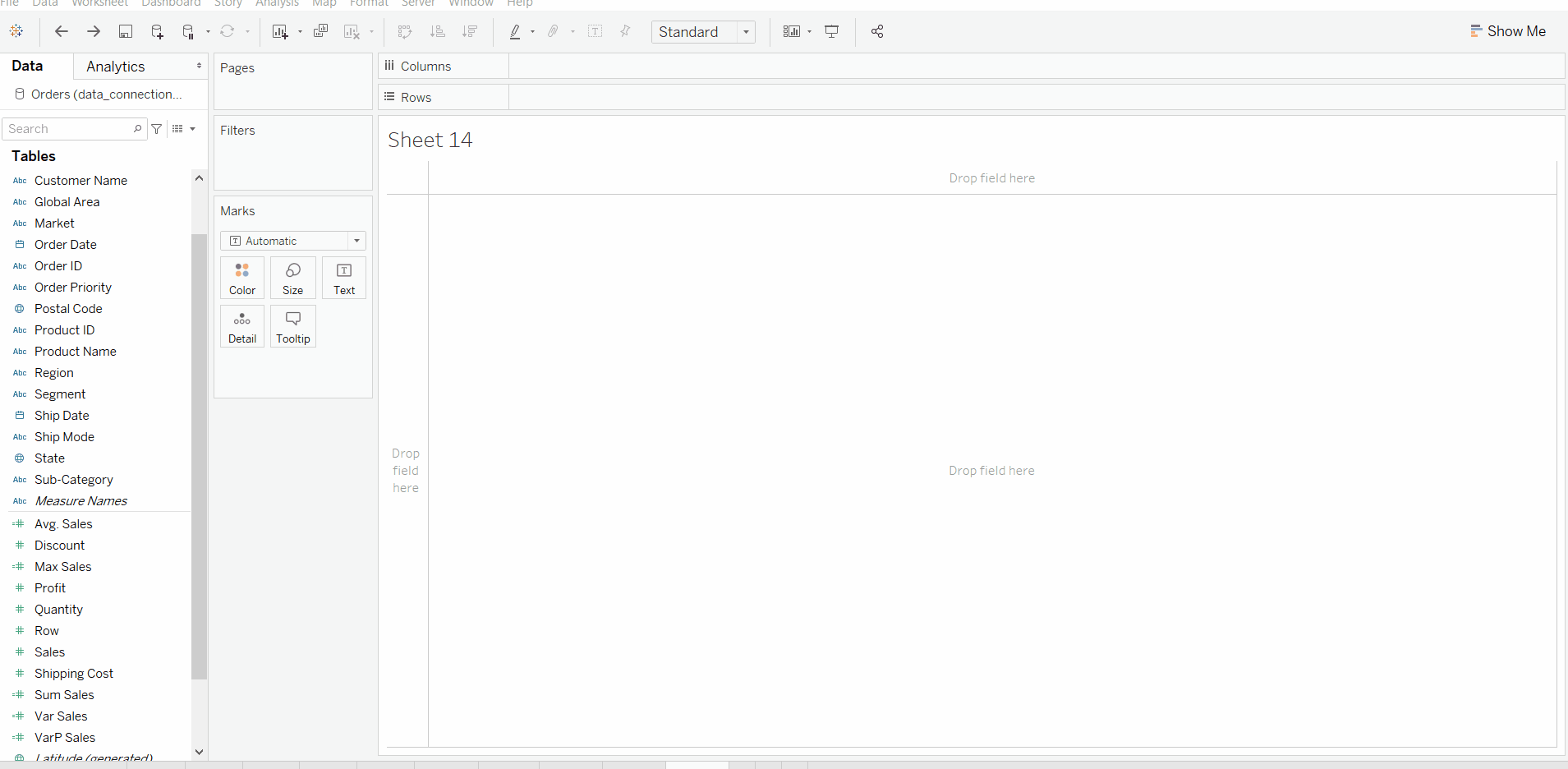### Tableau STDEVP Function

The Tableau STDEVP function is for locating the quality deviation of the whole population.

Syntax:

```STDEVP(Expression)
```

Example: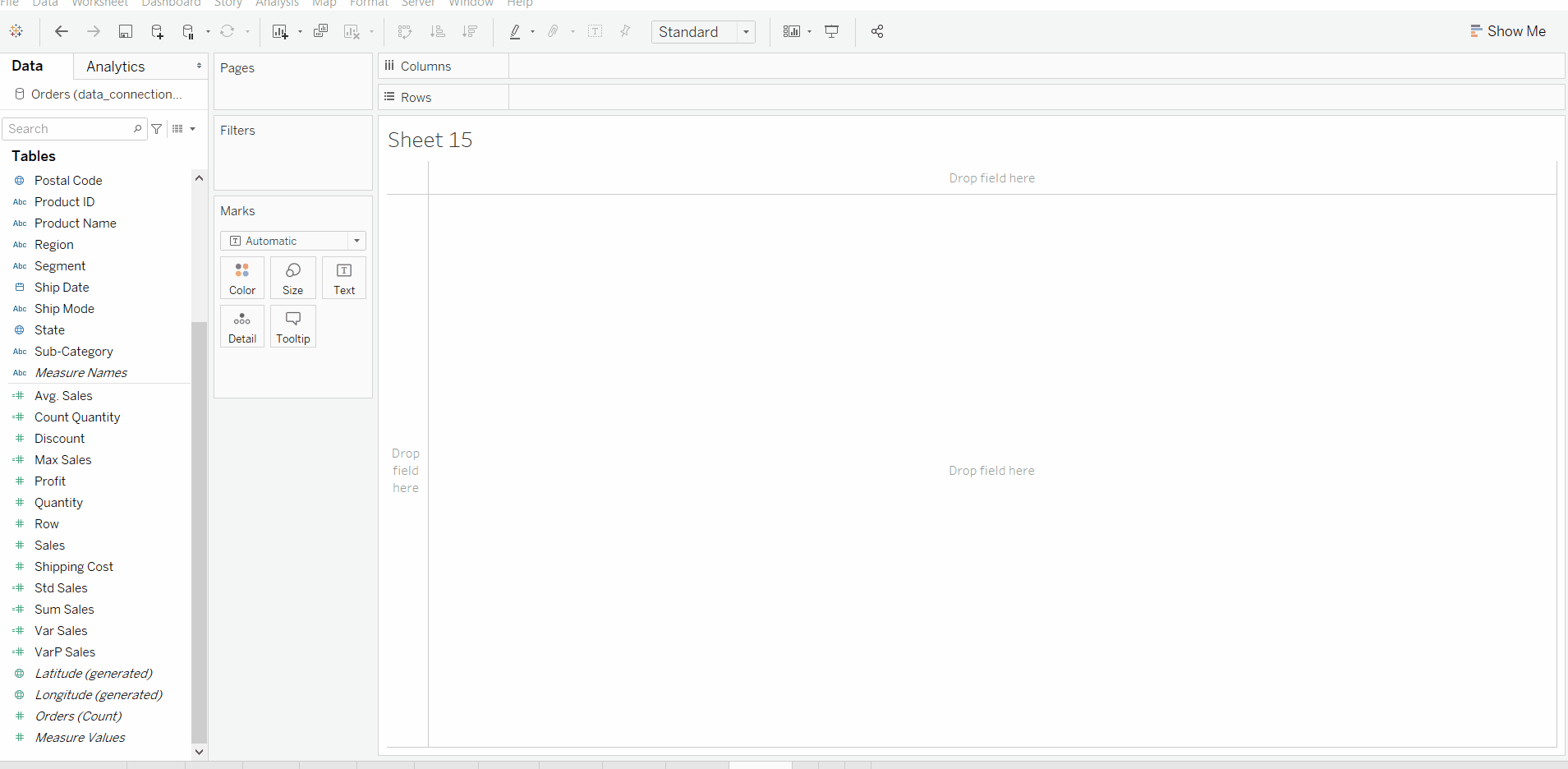### Tableau COUNT Function

The Tableau COUNT function is one of the Tableau aggregate function, which is used to find the number of not null values.

Syntax:

```COUNT(Expression)
```

Example: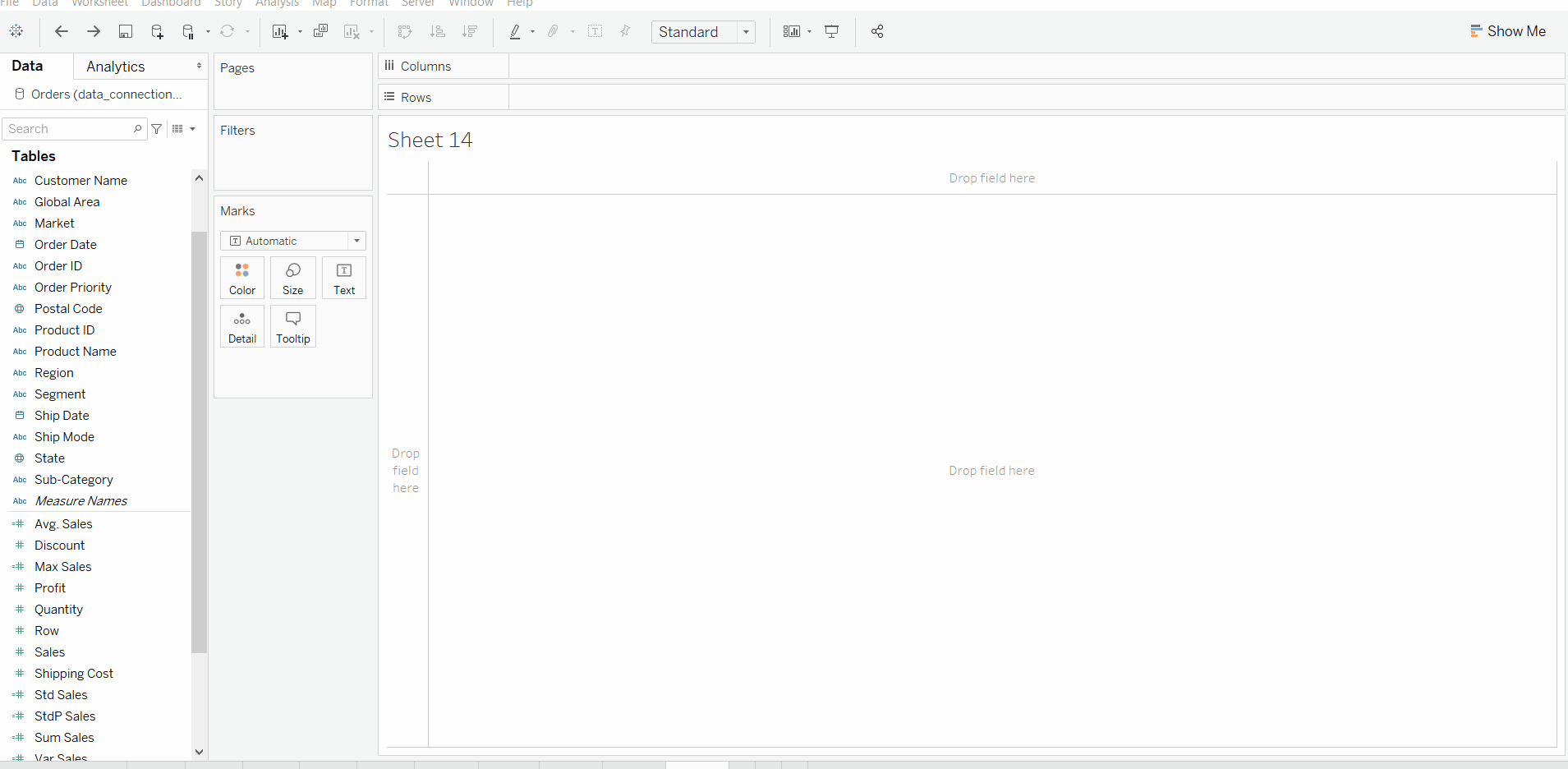TIP: Use COUNTD function to urge a definite count.

My Personal Notes arrow_drop_up